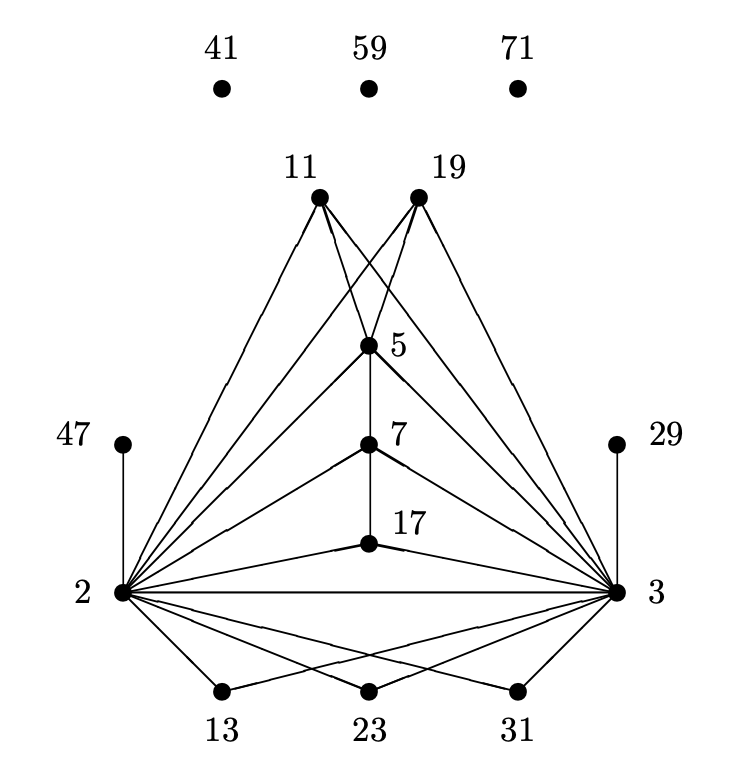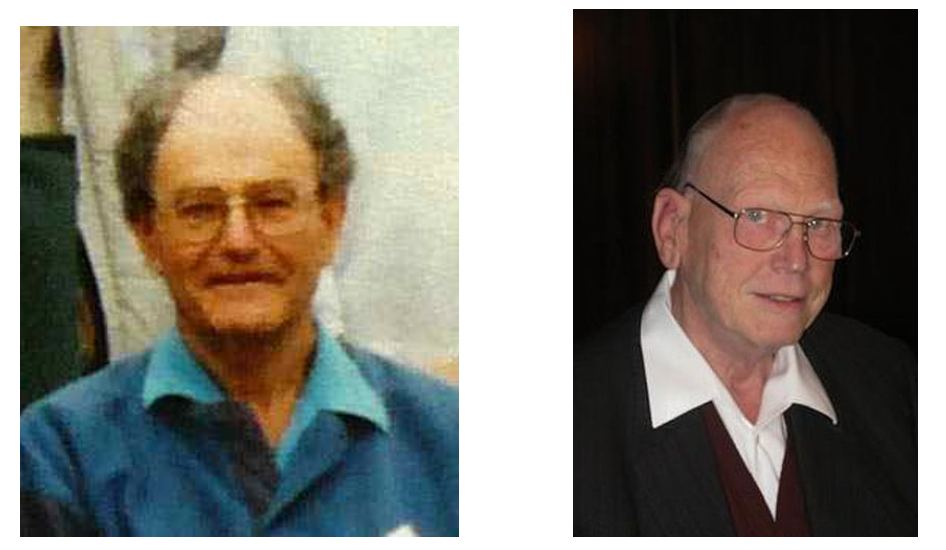# The monster prime graph

Here’s a nice, symmetric, labeled graph:The prime numbers labelling the vertices are exactly the prime divisors of the order of the largest sporadic group: the monster group $\mathbb{M}$.
$\# \mathbb{M} = 2^{46}.3^{20}.5^9.7^6.11^2.13^3.17.19.23.29.31.41.47.59.71$

Looking (for example) at the character table of the monster you can check that there is an edge between two primes $p$ and $q$ exactly when the monster has an element of order $p.q$.

Now the fun part: this graph characterises the Monster!

There is no other group $G$ having only elements of these prime orders, and only these edges for its elements of order $p.q$.

This was proved by Melissa Lee and Tomasz Popiel in $\mathbb{M}, \mathbb{B}$, and $\mathbf{Co}_1$ are recognisable by their prime graphs, by using modular character theory.

The proof for the Monster takes less than one page, so it’s clear that it builds on lots of previous results.

There’s the work of Mina Hagie The prime graph of a sporadic simple group, who used the classification of all finite simple groups to put heavy restrictions on possible groups $G$ having the same prime graph as a sporadic simple group.

For the Monster, she proved that if the prime graph of $G$ is that of the monster, then the Fitting subgroup $F(G)$ must be a $3$-group, and $G/F(G) \simeq \mathbb{M}$.

Her result, in turn, builds on the Gruenberg-Kegel theorem, after Karl Gruenberg and Otto Kegel.

The Gruenberg-Kegel theorem, which they never published (a write-up is in the paper Prime graph components of finite groups by Williams), shows the wealth of information contained in the prime graph of a finite group. For this reason, the prime graph is often called the Gruenberg-Kegel graph.The pictures above are taken from a talk by Peter Cameron, The Gruenberg-Kegel graph. Peter Cameron’s blog is an excellent source of information for all things relating groups and graphs.

The full proof of the Gruenberg-Kegel theorem is way too involved for a blogpost, but I should give you at least an idea of it, and of one of the recurrent tools involved, the structural results on Frobenius groups by John Thompson.

Here’s lemma 1.1 of the paper On connection between the structure of a finite group and the properties of its prime graph by A.V. Vasil’ev.

Lemma: If $1 \triangleleft K \triangleleft H \triangleleft G$ is a series of normal subgroups, and if we have primes $p$ dividing the order of $K$, $q$ dividing the order of $H/K$, and $r$ dividing the order of $G/H$, then there is at least one edge among these three vertices in the prime graph of $G$.

Okay, let’s suppose there’s a counterexample $G$, and take one of minimal order. Let $P$ be a Sylow $p$-subgroup of $K$, and $N$ its normaliser in $G$. By the Frattini argument $G=K.N$ and so $G/K \simeq N/(N \cap K)$.

Then there’s a normal series $1 \triangleleft P \triangleleft (N \cap H)=N_H(P) \triangleleft N$, and by Frattini $H=K.(N \cap H)$. But then, $N/(N \cap H)=H.N/H = G/H$ and $(N \cap H)/P$ maps onto $(N \cap H)/(N \cap H \cap K) = H/K$, so this series satisfies the conditions for the three primes $p,q$ and $r$.

But as there is no edge among $p,q$ and $r$ in the prime graph of $G$, there can be no such edge in the prime graph of $N$, and $N$ would be a counterexample of smaller order, unless $N=G$.

Oh, I should have said this before: if there is an edge between two primes in the prime graph of a subgroup (or a quotient) of $G$, then such as edge exists also in the prime group of $G$ (trivial for subgroups, use lifts of elements for quotients).

The only way out is that $N=G$, or that $P$ is a normal subgroup of $G$. Look at quotients $\overline{G}=G/P$ and $\overline{H}=H/P$, take a Sylow $q$-subgroup of $\overline{H}$ and $\overline{M}$ its normaliser in $\overline{G}$.

Frattini again gives $\overline{M}/(\overline{M} \cap \overline{H}) = \overline{G}/\overline{H}$, and $r$ is a prime divisor of the order of $\overline{M}/\overline{Q}$.

Lift the whole schmuck to the lift of $\overline{M}$ in $G$ and get a series of normal subgroups
$1 \triangleleft P \triangleleft Q \triangleleft M$
satisfying the three primes condition, so would give a smaller counter-example unless $M=G$ and $Q$ (the lift of $\overline{Q}$ to $G$) is a normal subgroup of $G$.

Sooner or later, in almost all proofs around the Gruenberg-Kegel result, a Frobenius group enters the picture.

Here, we take an element $x$ in $G$ of order $r$, and consider the subgroup $F$ generated by $Q$ and $x$. The action of $x$ on $Q$ by conjugation is fixed-point free (if not, $G$ would have elements of order $p.r$ or $q.r$ and there is no edge between these prime vertices by assumption).

But then, $F$ is a semi-direct product $Q \rtimes \langle x \rangle$, and again because $G$ has no elements of order $p.r$ nor $q.r$ we have:

• the centraliser-subgroup in $F$ of any non-identity element in $\langle x \rangle$ is contained in $\langle x \rangle$
• the centraliser-subgroup in $F$ of any non-identity element in $Q$ is contained in $Q$

So, $F$ is a Frobenius group with ‘Frobenius kernel’ $Q$. Thompson proved that the Frobenius kernel is a nilpotent group, so a product of its Sylow-subgroups. But then, $Q$ (and therefore $G$) contains an element of order $p.q$, done.

### Similar Posts:

This site uses Akismet to reduce spam. Learn how your comment data is processed.